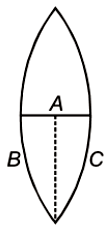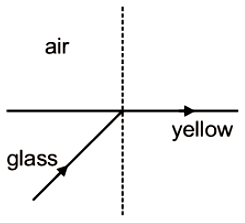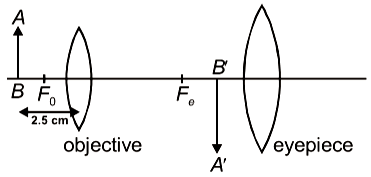A converging beam of light is incident on a concave lens of focal length 30cm, in such a way that their point of intersection would lie at a height 1cm above the principal axis and at a distance of 24cm from the position of the lens if there was no lens. Find the nature of the image formed when the beam passes through the lens.

1.  Real at a distance 60cm from the lens

2.  Real at a distance 120cm from the lens

3.  Virtual at a distance 60cm from the lens

4.  Virtual at a distance 120 cm from the lens

Concept Questions :-

Lenses
High Yielding Test Series + Question Bank - NEET 2020

Difficulty Level:

One face of a prism of apex angle 30$°$ is silvered and a ray of light is incident on the first face at an angle i such that it retraces its entire path after reflection from the silvered face. If the refractive index of prism material is 2, then the value of i must be -

1.  45$°$

2.  60$°$

3.  30$°$

4.  90$°$

Concept Questions :-

Prisms
High Yielding Test Series + Question Bank - NEET 2020

Difficulty Level:

The focal length of a convex lens is f. An object is placed at a distance x from the focal point and a real image is formed. The linear magnification has a magnitude

1.

2.

3.

4.  $\frac{x}{f}$

Concept Questions :-

Lenses
High Yielding Test Series + Question Bank - NEET 2020

Difficulty Level:

Which of the following is not due to the total internal reflection of light

1.  Brilliance of diamond

2.  Mirage on hot summer days

3.  Working of optional fiber

4.  formation of rainbow

Concept Questions :-

Total internal reflection
High Yielding Test Series + Question Bank - NEET 2020

Difficulty Level:

A ray of light incident on an equilateral prism at grazing incidence emerges from the prism at grazing emergence. Refractive index of the prism is

(1) 1.5

(2) 1.8

(3) 1.33

(4) 2

Concept Questions :-

Total internal reflection
High Yielding Test Series + Question Bank - NEET 2020

Difficulty Level:

The refractive index of the material of the prism for violet colour is 1.69 and that for red is 1.65. If the refractive index for mean colour is 1.66, the dispersive power of the material of the prism

1.  0.66

2.  0.06

3.  0.65

4.  0.69

Concept Questions :-

Prisms
High Yielding Test Series + Question Bank - NEET 2020

Difficulty Level:

Absolute refractive indices of glass and water are 3/2 and 4/3. The ratio of velocity of light in glass and water will be

1.  4:3

2.  8:7

3.  8:9

4.  3:4

Concept Questions :-

Refraction at plane surface
High Yielding Test Series + Question Bank - NEET 2020

Difficulty Level:

A thin equiconvex lens of power P is cut into three parts A, B, and C as shown in the figure. If  are powers of the three parts respectively, then1.

2.

3.

4.

Concept Questions :-

Lenses
High Yielding Test Series + Question Bank - NEET 2020

Difficulty Level:

A light beam containing red, yellow and blue color, falls on the interface of glass air as shown. If the yellow light is just totally internally reflected, then emergent ray in air contains-1.  Both red and blue color

2.  Only red color

3.  Only blue color

4.  No color

Concept Questions :-

Total internal reflection
High Yielding Test Series + Question Bank - NEET 2020

Difficulty Level:

Focal lengths of objective and eyepiece of a compound microscope are 2 cm and 6.25 cm respectively. An object AB is placed at a distance of 2.5 cm from the objective which forms the image B'A' as shown in the figure. Maximum magnifying power in this case is :1.  10

2.  20

3.  5

4.  25

Concept Questions :-

Simple and compound microscope